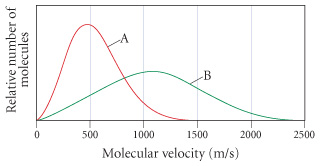# Problem: The following graph shows the distribution of molecular velocities for two different molecules (A and B) at the same temperature. Which molecule would have the higher rate of effusion? a) A b) B c) not enough information to determine

###### FREE Expert Solution

rate of effusion → proportional to molecular velocity

86% (387 ratings)###### Problem Details

The following graph shows the distribution of molecular velocities for two different molecules (A and B) at the same temperature. Which molecule would have the higher rate of effusion?a) A

b) B

c) not enough information to determine

Frequently Asked Questions

What scientific concept do you need to know in order to solve this problem?

Our tutors have indicated that to solve this problem you will need to apply the Velocity Distributions concept. You can view video lessons to learn Velocity Distributions. Or if you need more Velocity Distributions practice, you can also practice Velocity Distributions practice problems.

What professor is this problem relevant for?

Based on our data, we think this problem is relevant for Professor Voznyy's class at TORONTO.